Miscellaneous

Chapter 1 Class 11 Sets
Serial order wise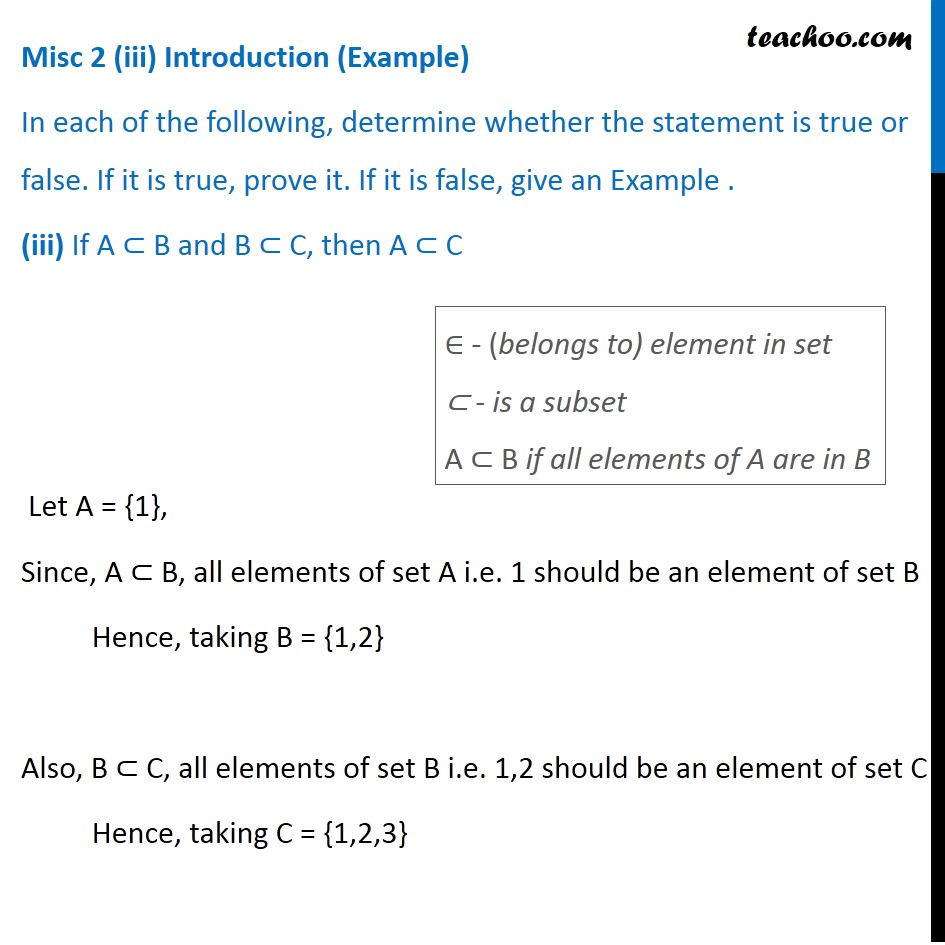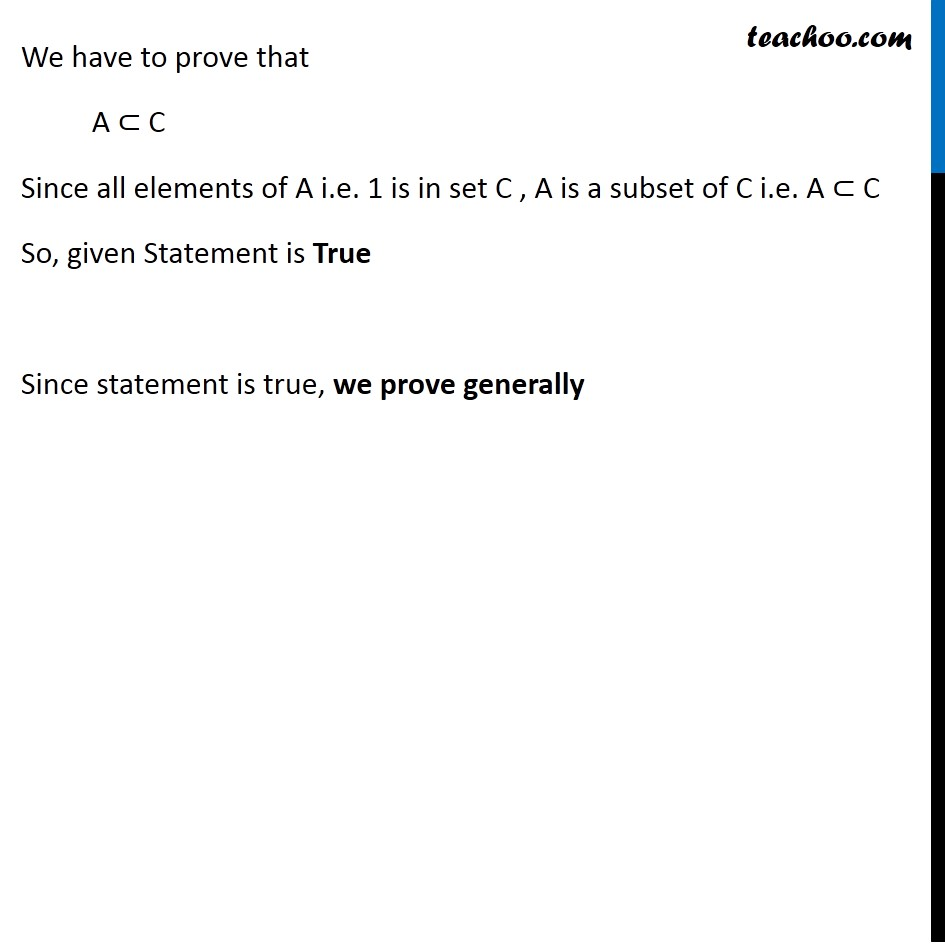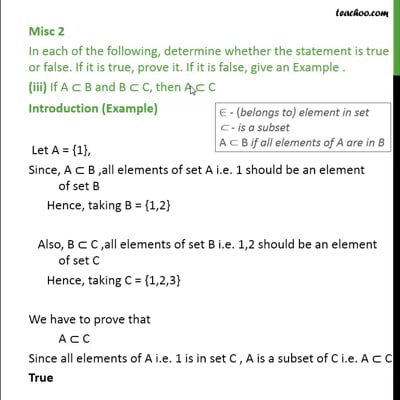This video is only available for Teachoo black users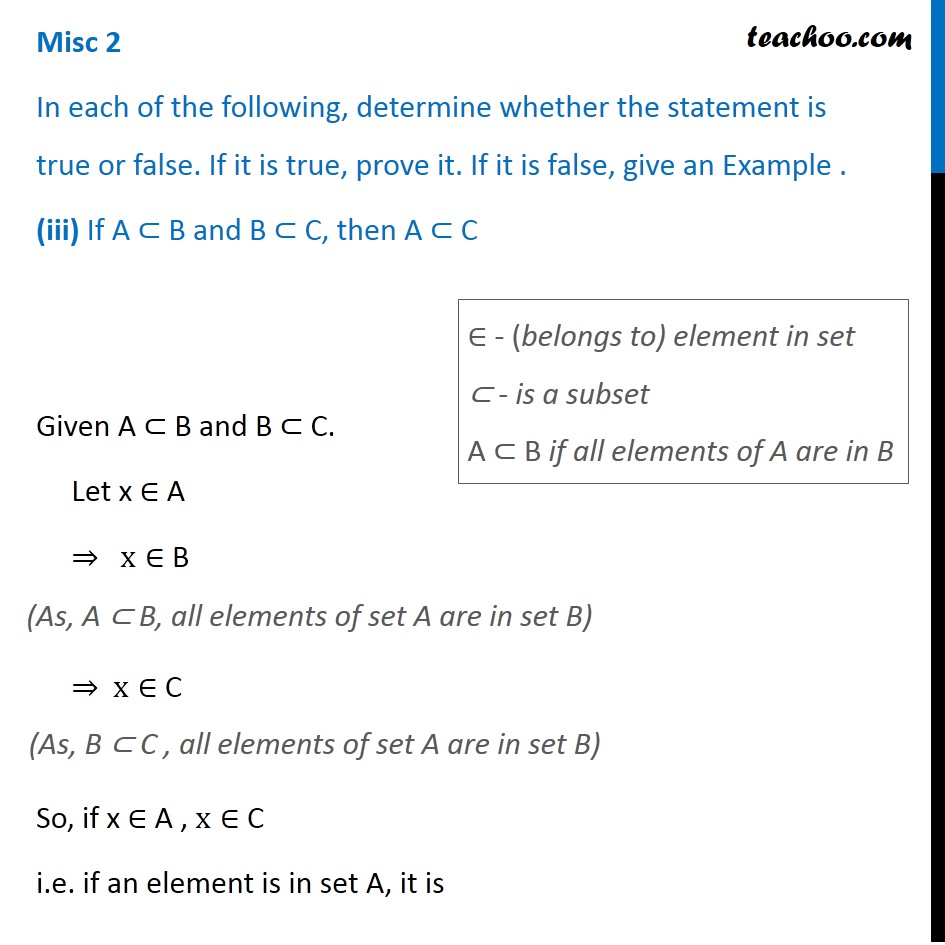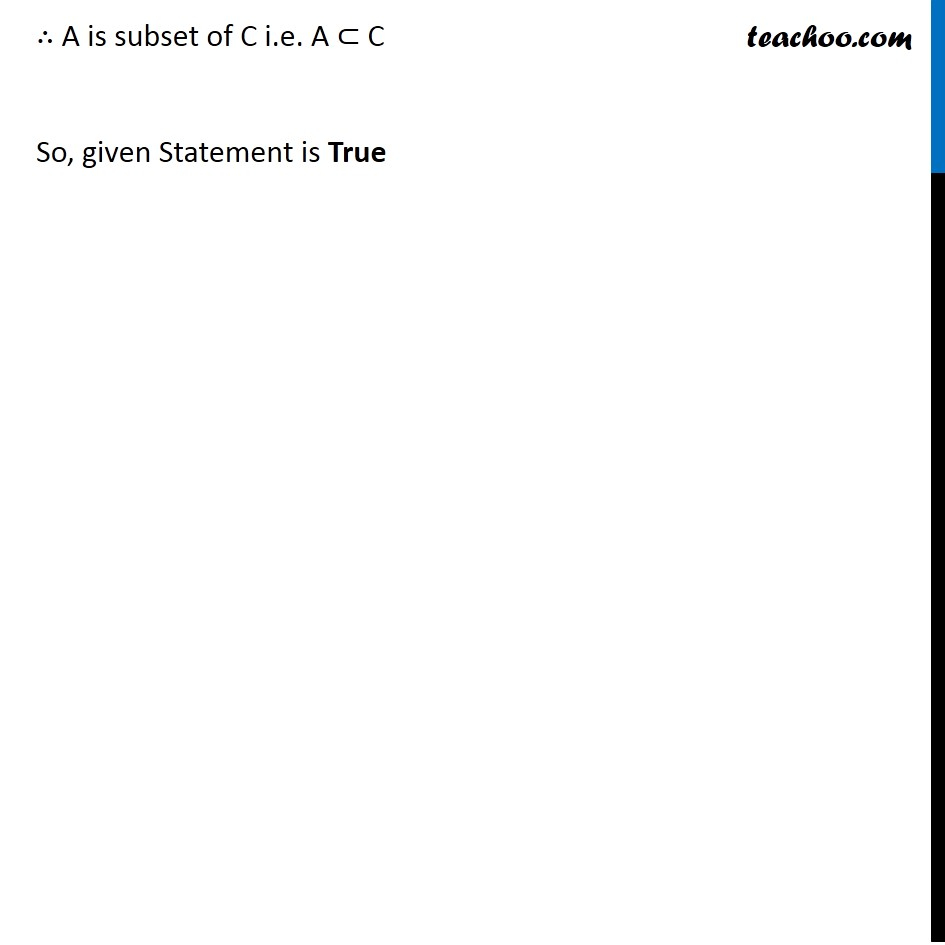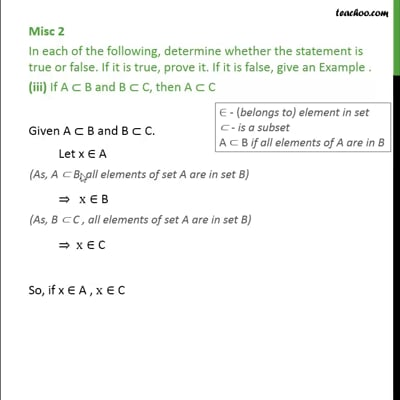This video is only available for Teachoo black users

Introducing your new favourite teacher - Teachoo Black, at only ₹83 per month

### Transcript

Misc 2 (iii) Introduction (Example) In each of the following, determine whether the statement is true or false. If it is true, prove it. If it is false, give an Example . (iii) If A ⊂ B and B ⊂ C, then A ⊂ C Let A = {1}, Since, A ⊂ B, all elements of set A i.e. 1 should be an element of set B Hence, taking B = {1,2} Also, B ⊂ C, all elements of set B i.e. 1,2 should be an element of set C Hence, taking C = {1,2,3} ∈ - (belongs to) element in set ⊂ - is a subset A ⊂ B if all elements of A are in B We have to prove that A ⊂ C Since all elements of A i.e. 1 is in set C , A is a subset of C i.e. A ⊂ C So, given Statement is True Since statement is true, we prove generally Misc 2 In each of the following, determine whether the statement is true or false. If it is true, prove it. If it is false, give an Example . (iii) If A ⊂ B and B ⊂ C, then A ⊂ C Given A ⊂ B and B ⊂ C. Let x ∈ A ⇒ x∈ B ⇒ x∈ C So, if x ∈ A , x∈ C i.e. if an element is in set A, it is ∈ - (belongs to) element in set ⊂ - is a subset A ⊂ B if all elements of A are in B ∴ A is subset of C i.e. A ⊂ C So, given Statement is True